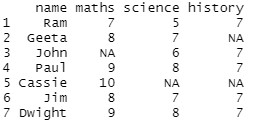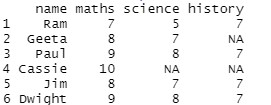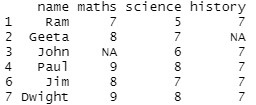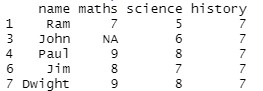Remove rows with NA in one column of R DataFrame

• Last Updated : 01 Apr, 2021

Columns of DataFrame in R Programming Language can have empty values represented by NA. In this article, we are going to see how to remove rows with NA in one column. We will see various approaches to remove rows with NA values.

Data in use:Approach

• Create a data frame
• Select the column on the basis of which rows are to be removed
• Traverse the column searching for na values
• Select rows
• Delete such rows using a specific method

Method 1: Using drop_na()

drop_na() Drops rows having values equal to NA. To use this approach we need to use “tidyr” library, which can be installed.

install.packages(“tidyverse”)

Syntax:

drop_na(name_of_the_column)

Example:

R

 # Creating dataframestudent=data.frame(name=c("Ram","Geeta","John","Paul",                          "Cassie","Jim","Dwight")                   ,maths=c(7,8,NA,9,10,8,9)                   ,science=c(5,7,6,8,NA,7,8)                   ,history=c(7,NA,7,7,NA,7,7))  print(student)  library(tidyr)student %>% drop_na(maths)

Output:Method 2: Using is.na()

is.na() function first looks for na values in a column and then discards such rows.

Syntax:

is.na(name of the column)

Example:

R

 # Creating dataframestudent=data.frame(name=c("Ram","Geeta","John","Paul",                          "Cassie","Jim","Dwight")                   ,maths=c(7,8,NA,9,10,8,9)                   ,science=c(5,7,6,8,NA,7,8)                   ,history=c(7,NA,7,7,NA,7,7))  print(student)  student[!is.na(student\$science),]

Output:Method 3:Using complete.cases()

This function functions similar to the above two methods

Syntax:

complete.cases(name of the column)

Example:

R

 # Creating dataframestudent=data.frame(name=c("Ram","Geeta","John","Paul",                          "Cassie","Jim","Dwight")                   ,maths=c(7,8,NA,9,10,8,9)                   ,science=c(5,7,6,8,NA,7,8)                   ,history=c(7,NA,7,7,NA,7,7))  print(student)  student[complete.cases(student\$history),]

Output:My Personal Notes arrow_drop_up